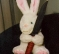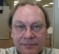## Differential frequency measurementStarted by 6 years ago12 replieslatest reply 6 years ago269 views

Hi all:

Can anyone suggest a good way of doing DTMF-like tone detection by determining the (known) frequency difference between limited time duration tone-pairs? Channel impairments and sampling rate errors make it difficult in this case to directly measure the tone frequencies, but the differences in tone pair frequencies are fixed. Using a brute force technique with FFTs seems obvious, but I'm concerned that the limited number of time-domain sample might not allow sufficient frequency domain resolution for this to work.

Thank you,

[ - ]A few questions:

1) How many wavelengths are in each short duration?

2) Are they consistent?

3) Is the sampling rate somewhat steady?

Here is an approach which may work based on this equation:

cos(A) + cos(B) = 2 cos[(A+B)/2] cos[(A-B)/2]

Find the zero crossings.  You should be able to get a good estimate of (A+B)/2 from these.  Build a signal by sampling at the midpoints between the zero crossings and alternating the values +/-.  You should be able to get a good estimate of (A-B)/2 from these.  Since you know the actual (A-B)/2, you should be able to get a good estimate of the sampling rate.  From there you can get the actual (A+B)/2.  You now know A+B and A-B so finding A and B become trivial.

This should work because most of the zero crossings are where cos[(A+B)/2] is zero.  At their midpoints cos[(A+B)/2] alternates between 1 and -1.

I haven't actually tried this, so good luck.

Ced
[ - ]Hello Ced:

1. Just like DTMF, there are two tones in each period

2. The tones are transmitted with good precison (< 0.1% in frequency), but are subject to phase/frequency distortion due to the channel, and sampling rate errors.

3. The sampling rate is relatively stable, but inaccurate (PC sound card)

Thanks!

[ - ]A key assumption of the approach I mentioned above is that the two tones are of the same amplitude.  If they aren't, the summation equation becomes considerably more complicated.  It is still doable in the time domain though.

It isn't quite clear to me what your overall objective is though.  Are your frequencies from a pre-defined set of values like DTMF?  If that is the case, then the inaccuracy of a PC sound card should be irrelevant.  On the other hand, if you are trying to find the precise values of the two frequencies from a varying range, then the precision of the sampling card being of concern to you means you must be trying to find the frequency value to even a greater precision.

I'm also curious about how much distortion/noise is introduced by your transmission channel.

I'm inclined to think that a DFT approach would be superior.  With only two tones, a small number of sample points is not going to be a problem.  You don't have to calculate the entire DFT, only the bins within the range of interest.

If this is for a commercial application I would be happy to code a solution for you for a reasonable fee.  You can email me directly to cedron at exede dot net.

Ced
[ - ]PC sound cards use 100 ppm or better crystals. That is 10 times more accurate than your stated 0.1% transmit accuracy.

You earlier stated "..but are subject to phase/frequency distortion due to the channel..". If this is via radio waves or other linear medium, frequency corruption is not possible. Even nonlinear mediums cannot change frequency, but they can create harmonics of any sinusoids in that medium due to compression, clipping, zero-cross distortion, etc. If sent by radio, then any relative motion between transmitter and receiver will have Doppler effects that can change the frequency! If transmission is via POTS (plain old telephone service), a modern POTS is very accurate (unless it's a third world country maybe). Packet assembly/disassembly may still have segments of dropouts due to network timing constraints. What's not typically good in POTS is amplitude flatness over frequency, but that's so much better than it was back in the Ma Bell days since everything is digitized and stays digital in the network.

If this is being done on a PC, then it's been my experience that it's the PC's inability to provide 100% CPU and data bus access to avoid internal dropouts (e.g. dropped samples). You'd be better off using something like a Teensy that may not have the same processing power of an Intel or AMD PC processor, but at least it's CPU availability is 100%, if the code is written correctly.

Any good solution starts with a good analysis of the system under test. Everything must be stated concisely and precisely as possible, or there won't be enough information to create a solution.

[ - ]Hi artmez:

The real problem with frequency accuracy in this system is that it is designed to use AM modulation over HF radio links, but in this case, the receiver is a SSB receiver. In theory, it should be possible to make this work if the receiver is tuned precisely to (and remains at) the transmitter's carrier frequency, but in practice, most of the frequency error will result from carrier offset errors in the SSB receiver. I agree that the PC soundcard sampling rate errors will typically be trivial compared to this. As you mention, relative motion between the transmitter and receiver can affect the frequency, and in this case, ionospheric propagation at HF is well known for imparting small doppler shifts. However, I think these are also probably typically small compared to the receiver frequency errors.

Thanks,

[ - ]So there are 3 sources of frequency and phase error:  the original modulation signal,  the SSB carrier generator (which could be reduced if it was synchronized with the modulation source generation), and  the SSB demodulation. Even so, all of that should be small compared to the what I expect to be a slow data rate since you are using dual-tones, but you have not provided enough details regarding that other than "like DTMF", which implies it is not DTMF.

Maybe tackle this from another perspective. I'm guessing the concern is the accuracy of the underlying data, i.e. the BER. BER is statistical and depends on the underlying error distribution, but in general, there can be high peak errors within any small period. If the data channel is noisy, which includes the entire end-to-end process, one way to mitigate that is add FEC (forward error correction) to the data stream. The tradeoff is complexity vs. data throughput vs. accuracy. It's like Heisenberg's uncertainty principle in a sense -- optimizing one is done at the expense of the others. The choice of FEC depends on the channel's error properties (e.g. burst vs. single bit errors). So for example, it may be as simple as adding 4 bits of Hamming correction to every 4 bits of data (halving the throughput of course) and then performing single bit error correction and double bit error detection with a simple 16 byte lookup table (as I did in my first data communications job back in 1980).

Another approach is like what IBM pioneered when their hard disk driver bit density approached the theoretical limit for flux transitions relative to the area occupied on the disk's surface (before commercializing vertical recording) --  they used a maximum-likelihood algorithm that did an "intelligent" guess of what the bit was based on what effectively was the channel's error characteristics. Older telco modems did something similar when they started including channel equalization in modem algorithms (which was based on the underlying, mostly analog methods of POTS operation). These include methods of detecting the underlying error rate and then "retraining" (changing the equalization) for the channel's changing noise characteristics.

Is the signal generation and detection design locked in and you are looking for a way to improve performance? This still could be to add FEC to the data stream, unless there are other constraints preventing that. Otherwise, it may by that you have painted yourself into a corner.

[ - ]Hi artmez:

The signal generation is definitely out of my control. The detection design is what I am focused on. Other designs for this system that use an (external) SSB receiver and a PC soundcard have mediocre accuracy and sensitivity. I have written a matched filter detector in Python, and it has similar problems when using a SSB receiver, presumably due to the frequency offset errors I mentioned previously. If I use an AM receiver, then the matched filter detector performs well. The constraint for this design is that it must use a SSB receiver, and that is why I have been thinking about the differential frequency approach to detecting the tone pairs (each pair of tones has a unique difference between their frequencies).

Thanks,

[ - ]I whipped up a graphic to demonstrate the sum of two tones of the same amplitude.The top graph is the two tones cos(A) and cos(B).  The bottom graph has cos(A)+cos(B) in blue.  The envelope is 2*cos[(A-B)/2] in red.  The grey one is cos[(A+B)/2]/10.  The rescaling is to make the graphic less messy.

Ced
[ - ]I doubt if knowing the frequency difference would help you unless you have a priori knowledge of one of the tone frequencies.

The highest resolution spectrum estimation for a short fixed length of data is done using the MESA algorithm.  You can find the code in C+ in Numerical Recipes and in Python.

John

[ - ]Thank you John. Yes, in this case the transmitted tone frequencies (and hence the differences between them) are known a priori. What is not known is the precise sampling rate, and hence the exact frequencies of the tones in the input signal. However, the differences between these tones should be relatively constant.

[ - ]The problem seems simple if only the sample rate is unknown.  The ratio of the two tones is constant regardless of the error induced by the sample rate.  So go ahead and measure the tones, compute the ratio of their frequencies, and the ratio will give you the tone pair with which you are dealing.

[ - ]